# Process Tolerancing: A Solution To The Dilemma Of Worst-Case Versus Statistical Tolerancing

Dr. Wayne A. Taylor

When solving tolerancing problems, one must choose between worst-case tolerancing and statistical tolerancing.  Both of these methods have their pros and cons.  If worst-case tolerancing is used, all tolerances must be specified as worst-case tolerances. If statistical tolerancing is used, all tolerances must be specified as statistical tolerances.  In reality, the behavior of certain inputs is best described using worst-case tolerances while the behavior of other inputs is best described by statistical tolerances.  Still, other inputs are not adequately represented by either type of tolerance.  This article reviews the two current methods of tolerancing along with their pros and cons.  It then introduces a new method of tolerancing, called process tolerancing, which solves many of the problems associated with the current approaches.  Process tolerancing represents a unified approach to tolerancing that encompasses both of the previous approaches.  Using process tolerancing, worst-case tolerances and statistical tolerances can be combined into the same analysis.  The flexibility provided by process tolerancing results in solutions to such common problems as multiple components from the same lot and off-center processes.
KEYWORDS: Statistical Tolerance, Worst-Case Tolerance, Process Tolerance, Variation Transmission Analysis, Propagation of Error, and Six Sigma Quality.

INTRODUCTION

Tolerance analysis is used during product design. For example, consider the design of a pump.  Flow rate is a key characteristic that must be controlled. It is called an output variable.  Flow rate is affected by such factors as the diameter of the piston, stroke length, motor speed and viscosity of the solution.  These factors are called input variables.  The goal of tolerance analysis is to predict the behavior of the output variable based on an understanding of how the inputs behave.  The behavior of the inputs can then be adjusted to cause the output to behave as desired.

Tolerance analysis is equally important to process development. For example, consider the installation of a new heat seal machine.  Seal strength is the output variable.  Input variables include die temperature, seal time, and pressure.  Using tolerance analysis, a process window can be determined for the inputs that ensures that seal strength stays within its specification limits.

Two methods of tolerancing currently exist: worst-case and statistical tolerancing.  Both have their pros and cons.  These two methods will be reviewed and compared.  A new method of tolerancing, called process tolerancing, will then be introduced.  Process tolerancing represents a unified approach to tolerancing that encompasses both the previous approaches.

The greatest benefit of process tolerancing is that it allows both worst-case and statistical tolerances to be combined in the same analysis.  Some inputs are best described by worst-case tolerances.  For example, consider solution viscosity for the pump.  Different customers will use the pump with different solutions.  Each customer wants the correct flow rate.  As a result, a worst-case tolerance best describes the behavior of solution viscosity.  Some inputs are best described by statistical tolerances.  For example, consider die temperature on the heat seal machine.  Die temperature is controlled automatically using closed-loop feedback.  This ensures it remains at the specified target.  Its behavior is best described using a statistical tolerance.  The purpose of a tolerance analysis is to predict the behavior of the output based on knowledge of how the inputs behave.  Key to its success is the ability to accurately describe the behavior of the inputs.  Using process tolerancing, you can select the type of tolerance that best describes the behavior of each input.

Some inputs are not well represented by either worst-case or statistical tolerances.  For example, inputs from processes that are off-center or lack statistical control.  Process tolerancing offers much greater flexibility than the current two methods and can be used to better represent such situations.  Because of this added flexibility, process tolerancing provides solutions to such common problems as multiple components from the same lot and off-center processes.

WORST-CASE TOLERANCES

Worst-case tolerances are specified in the form 1.00 ± 0.03″.  The tolerance half-width of 0.03″ represents the maximum amount that a “good” unit can deviate from target.  This worst-case tolerance can also be represented in interval form as (0.97″, 1.03″).  A unit falling outside the range of 0.97″ and 1.03″ fails to meet the tolerance and is called a nonconformance or defect.  With worst-case tolerances, units of product can fall anywhere in the tolerance interval.  All units could be 0.97″, all units could be 1.03″, or the units could be uniformly spread across the interval.  Nonsymmetrical tolerances can also be specified, but are ignored here because they can be easily translated into equivalent symmetrical tolerances.

If worst-case tolerances are specified for the inputs, a worst-case tolerance can be calculated for the output.  Let the output be represented by y and the inputs by x1, …, xn.  Suppose y = f(x1, …, xn) and that the inputs have worst-case tolerances txi ± D xi.  Then the resulting worst-case tolerance for y, denoted ty ± D y, is: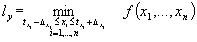(Eq. 1)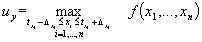(Eq. 2)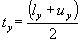(Eq. 3)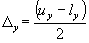(Eq. 4)

The terms ly and uy represent the minimum and maximum values of y over the worst-case tolerances for the inputs.  The worst-case tolerance for y is the interval (lyuy).  The target for yty, is the midpoint of this interval while Dy is half the width of the interval.  The following example demonstrates the use of these equations.

Example: Suppose that four identical components are stacked on top of each other and that we are interested in controlling the overall height.  Overall height is denoted by h.  Specification limits for overall height are from 3.9″ to 4.1″.  The height of the four components are denoted by d1, …, d4.  Suppose these components have a tolerance of 1.00 ± 0.03″.  Then: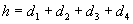(Eq. 5)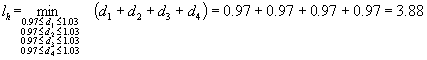(Eq. 6)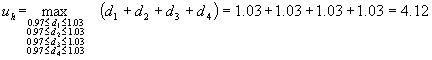(Eq. 7)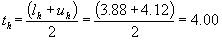(Eq. 8)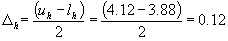(Eq. 9)

The resulting worst-case tolerance for h is 4.00 ± 0.12″ or (3.88″, 4.12″).  This tolerance is shown in Figure 1 along with the specification limits.  Worst-case tolerancing, predicts that the specification limits will not be meet and that the tolerance for component height must be tightened.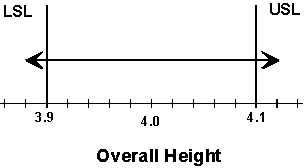Figure 1: Worst-case tolerance for overall height
4-component stack example

When the output is the sum of the inputs as in the above example, the worst-case tolerance equations simplify to: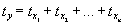(Eq. 10)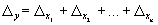(Eq. 11)

Equation 11 is frequently cited in textbooks on tolerancing.  However, when more complex equations are involved, Equations 1-4 must be used instead.

STATISTICAL TOLERANCES
Statistical tolerances are determined by a target, tx, and maximum standard deviation, sx.  In this article, they are denoted by tx £ sx.  They are more commonly denoted by tx ± D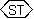.  When using thesymbol, it is most common to have D x = 3sx.  However, this relationship is not formalized in the standards and the six sigma approach uses D = 6sx.  Throughout this article, we will assume that D x = 3sx.  Using this interpretation, both 1.00 £ 0.01″ and 1.00 ± 0.03″denote the statistical tolerance with target tx = 1.00″ and maximum standard deviation sx = 0.01″.

The worst-case tolerance 1.00 ± 0.03″ and the statistical tolerance 1.00 ± 0.03″both assume that units of product fall in the interval (0.97″, 1.03″).  However, the statistical tolerance is more restrictive.  The statistical tolerance further requires that the units of product have an average of 1.00″ and a standard deviation no greater than 0.01″.  Statistical tolerancing assumes centered processes.  It further assumes that the inputs vary independent of each other, i.e., are not correlated.  Figure 2 shows a production batch that meets the worst-case tolerance 1.00 ± 0.03″ but fails to meet the assumptions of the corresponding statistical tolerance 1.00 ± 0.03″.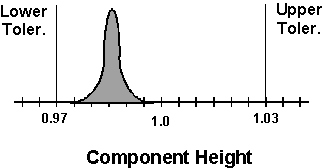Figure 2: Production batch satisfying the requirements of a
worst-case tolerance but not a statistical tolerance

If statistical tolerances are specified for the inputs, a statistical tolerance can be calculated for the output.  This amounts to determining the average and standard deviation of the output.  Simulations can always be used to predict these two values.  However, there also exist a variety of methods for deriving approximate and sometimes exact equations for the average and standard deviation.  Details can be found in Taylor (1991), Cox (1986) and Evans (1975).  These approaches have a variety of names including statistical tolerance analysis, propagation of errors and variation transmission analysis.

Returning to the case where y = f(x1, …, xn), equations can be derived for the average and standard deviation of y in terms of the average and standard deviation of the xi’s.  These will be denoted m y (m x1, …, m xn, s x1, …, s xn) and s y (m x1, …, m xn, s x1, …, s xn) respectively.  The statistical tolerance for y can then be calculated as follows: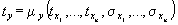(Eq. 12)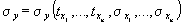(Eq. 13)

When the output is the sum of the inputs, the statistical tolerancing equations simplify to: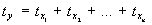(Eq. 14)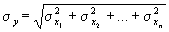(Eq. 15)

Multiplying both sides of Equation 15 by 3 gives: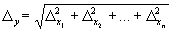(Eq. 16)

Equation 16 is frequently cited in textbooks on tolerancing.  It still holds even if we assume D = 6sx.  When more complex equations are involved, Equations 12 and 13 should be used instead.

Example (continued): Returning to the example of four components stacked on top of each other, assume each component has a statistical tolerance of 1.00 ≤ 0.01″.  Then the statistical tolerance for overall height is: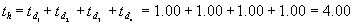(Eq. 17)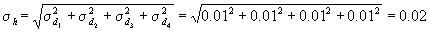(Eq. 18)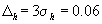(Eq. 19)

The resulting statistical tolerance for h is 4.00 ≤ 0.02″ or (3.94″, 4.06″). This tolerance is shown in Figure 3 along with the specification limits. Statistical tolerancing predicts that the specification limits will be easily met. No change is required to the tolerance for component height.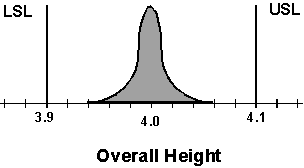Figure 3: Statistical tolerance for overall height
4-component stack example

COMPARISON OF THE TWO APPROACHES TO TOLERANCING

In the 4 component stack example, worst case tolerancing results in a tolerance for overall height of 4.00 ± 0.12″ while statistical tolerancing results in a tolerance of 4.00 ± 0.06″.  The worst-case tolerance is double the width of the statistical tolerance.  Statistical tolerancing predicts that the specification limits will be met and that the tolerance for component height does not need to be tightened.  Worst-case tolerancing predicts that the specification limits will not be met and that the tolerance for component height must be tightened.  The two methods of tolerancing produce different results and that can lead to opposite conclusions.  Selecting which method of tolerancing to use is an important decision.  This section examines the pros and cons of each approach.

Worst-case tolerancing is the safer approach.  If the inputs are within their respective tolerances, the output is guaranteed to be within its worst-case tolerance.  This is especially important for products like heart values or critical components on airplanes.  However, this guarantee comes at a high cost.  Worst-case tolerancing guards against a worst-case scenario that is highly unlikely if not impossible in most situations.  Worst-case tolerancing guards against all inputs simultaneously being at the same extreme.  In the 4-component stack example, this would require that all four components be either 0.97″ or 1.03″.  For this to occur repeatedly, would require the entire batch of components to be at one of the two extremes.  This could only occur if the process producing the components had zero variation.

Suppose instead that the process producing the components had a standard deviation of 0.005″.  As shown in Figure 4, the process average must stay between 0.985″ and 1.015″.  Otherwise, some components would fall outside the tolerance limits.  The two normal curves in Figure 4 represent the worst-case conditions for component height when the standard deviation is 0.005″.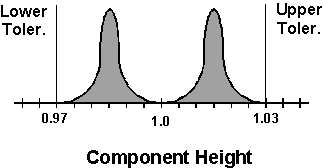Figure 4: Worst-case conditions for component height when s = 0.005″

When component height has an average of 0.985″ and a standard deviation is 0.005″, overall height will have an average of 3.94″ and a standard deviation of 0.0025″.  Likewise when component height has an average of 1.015″ and a standard deviation is 0.005″, overall height will have an average of 4.06″ and a standard deviation of 0.0025″.  These two sets of conditions represent the worst-case scenarios for overall height.  They are shown in Figure 5.  As a result of the variation, so long as the components meet their tolerance, overall height will easily meet the specification limits.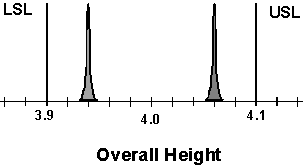Figure 5: Resulting worst-case conditions for overall height when s = 0.005″

Worst-case tolerancing generally overestimates the range of the outputs.  This can result in the unnecessary tightening of tolerances for the inputs, which drives up costs.  Worst-case tolerancing is the safer but more costly of the two approaches.

Statistical tolerancing results in narrower tolerances for the outputs.  In the 4-component stack example, statistical tolerancing resulted in a tolerance for overall height that was half as wide as that obtained using worst-case tolerancing.  As a result, the tolerances of the inputs do not have to be tightened as frequently or by as much.  Statistical tolerancing is the less costly of the two approaches.  When applicable, we would like to use statistical tolerancing.  However, statistical tolerancing assumes centered processes.  Violation of this assumption can result in defective product.

To see how let us return to the 4-component stack example.  Figure 6 shows how component height is suppose to behave when a statistical tolerance of 1.0 ± 0.03″is specified (m = 1.0″, s = 0.01″).  It also shows the corresponding behavior of overall height (m = 4.0″, s =0.02″).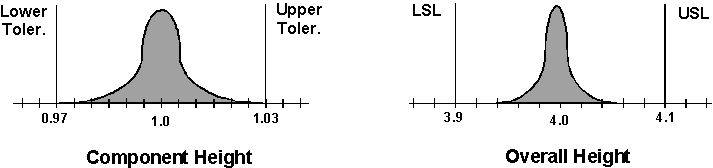Figure 6: How component height and overall height are suppose to behave

Consider what would happen if the component height actually behaved as in Figure 7 (m = 0.976″, s = 0.002″).  All the component heights are within the tolerance.  However, the process is off-center.  Figure 7 also shows the resulting behavior of overall height (m =3.904″, s = 0.004″).  As a result of the components being off-center, the overall height of some units exceeds the specification limits and all the units exceed the statistical tolerance of 3.94″ to 4.06″.  Statistical
tolerancing, while less costly, can underestimate the range of the outputs.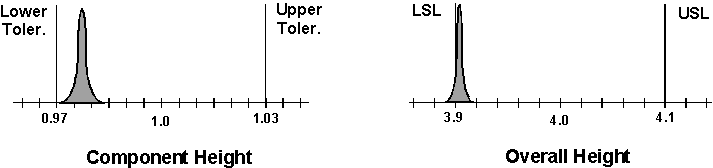Figure 7: How component height and overall height actually behave

To counter the tendency of statistical tolerances to underestimate the range of the outputs, Bender (1962) recommends multiplying statistical tolerances by a factor of 1.5.  For the 4-component stack example, this results in a tolerance of 4.0 ± 0.09″ or (3.91″, 4.09″).  The resulting tolerance is halfway between the statistical and worst-case tolerances. It is probably a more accurate estimate of the actual behavior of overall height.  However, the factor 1.5 is somewhat arbitrary and certainly not appropriate for every problem.

Another way of adjusting for the tendency of statistical tolerances to underestimate the range of the outputs is to estimate separately the amount that the average varies around the target and then calculate the total variation of the input as follows: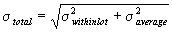Benderizing tolerances amounts to assuming that the process average has a standard deviation equal to 112% of that of the within-lot standard deviation.

These two approaches are only appropriate when the process varies around the target over longer periods of time.  Neither approach properly accounts for a process that is consistently run off-target.  For example, sheeting thickness might purposely be run close to the lower specification limit in order to reduce material usage.

THE DILEMMA

Statistical tolerancing is less costly.  We would like to use it when appropriate.  If statistical tolerancing is selected, statistical tolerances must be specified for all inputs.  However, statistical tolerances are not appropriate for all inputs.  To be safe, if some inputs are best represented by worst-case tolerances, worst-case tolerancing should be used instead.  But if worst-case tolerancing is selected, worst-case tolerances must be specified for all inputs, even those meeting the assumptions of statistical tolerancing.  This dives up the cost.  What is needed is a method of combining the two types of tolerances into a single analysis.  Process tolerancing, the topic of the next section will allow us to do just this.

PROCESS TOLERANCING

Process tolerancing is a new approach to tolerancing that is implemented in Taylor (1997).  A process tolerance consists of a minimum average, maximum average and maximum standard deviation. For example, it might be specified that the average for component height be between 0.99″ and 1.01″ and that the standard deviation be no more than 0.0067″.  This process tolerance is shown in Figure 8.  The two normal curves represent the minimum and maximum average with the maximum standard deviation.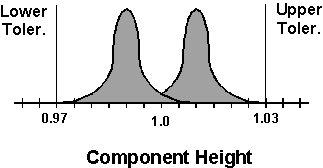Figure 8: Process tolerance for component height

Process tolerances will be denoted by tx ±D x £ s x.  The term tx ± D x denotes an interval containing the average. The minimum average is txD x and the maximum average is t+ D x.  The maximum standard deviation is s x.  For example, the process tolerance shown in Figure 8 is denoted by 1.0 ± 0.01 £0.0067″.  Process tolerances ensure that individual units all fall in the range (txD x-3s x, tx+D x+3x).  As shown in Figure 8, the process tolerance 1.0 ± 0.01 £ 0.0067″ ensures individual components fall in the interval (0.97″, 1.03″).

Process tolerances differ from worst-case tolerances in that worst-case tolerances represent requirements for individual components while process tolerances represent requirements for the process producing the components. Hence it’s name.  Process tolerances are more similar to statistical tolerances.  However, process tolerances do not require that the process remain centered. Instead, process tolerances specify an operating window that the process average must remain within.

If process tolerances are specified for the inputs, a process tolerance can be calculated for the output.  This requires equations for the average and standard deviation.  Returning to the case where y = f(x1, …, xn), let these two equations be represented by m y ( m x1, …, m xn, s x1, …, s xn) and s y (m x1,…, m xn,s x1,…, s xn).  These two equations can be obtained using the same methods used to calculate statistical tolerances.  Further, let the process tolerance for xi be denoted tx± D xi £ s xi.  Then the resulting process tolerance for y, denoted ty ± D y £ s y, is: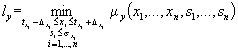(Eq. 21)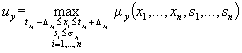(Eq. 22)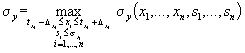(Eq. 23)(Eq. 24)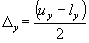(Eq. 25)

If the inputs satisfy their respective process tolerances, then y is guaranteed to satisfy the process tolerance given by Equations 21-25.

When the output is the sum of the inputs, the process tolerancing equations simplify to: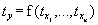(Eq. 26)(Eq. 27)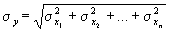(Eq. 28)

Equation 27 is the same as Equation 11 for worst-case tolerances.  Equation 28 is the same as Equation 15 for statistical tolerances.  A process tolerance represents a worst-case tolerance for the average and a statistical tolerance for the variation around the average.  When more complex equations are involved, Equations 21 through 25 should be used instead.

Example (continued): Returning to the example of four components stacked on top of each other, assume each component has a process tolerance of 1.00 ± 0.01 £ 0.00667″.  This tolerance was shown in Figure 8.  It is roughly equivalent to the worst-case tolerance 1.00 ± 0.03″ and the statistical tolerance 1.00 £ 0.01″.  All three tolerances result in units in the range (0.97″, 1.03″).  The resulting process tolerance for overall height is calculated as follows:(Eq. 29)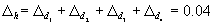(Eq. 30)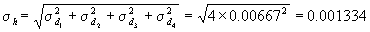(Eq. 31)

The resulting process tolerance for h is 4.00 ± 0.04£ 0.01334″.  This tolerance is shown in Figure 9 along with the specification limits. Individual units of product are expected to fall in the interval (3.92″, 4.08″).  Process tolerancing predicts that the specification limits will be met.  No change is required to the tolerance for component height.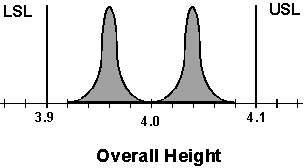Figure 9: Process tolerance for overall height
4-component stack example

The results of the three different approaches to tolerance analysis are summarized in Figure 10.  Process tolerancing predicts the overall heights will be in the interval (3.92″, 4.08″).  This falls between the worst-case tolerance of (3.88″, 4.12″) and the statistical tolerance of (3.94″, 4.06″).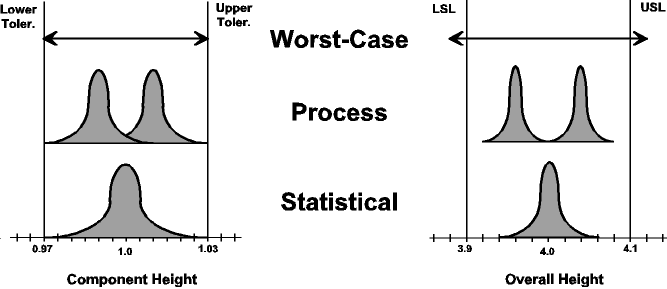Figure 10: Comparison of three approaches to tolerance analysis
4-component stack example

A UNIFIED APPROACH TO TOLERANCING

Process tolerancing represents a unified approach to tolerancing that encompasses both the previous approaches.  The process tolerance tx ±D x £ 0 is, in fact, a worst-case tolerance.  In this case, Equations 21-25 simplify to Equations 1-4.  The process tolerance tx ± 0 £s x is, in fact, a statistical tolerance.  In this case, Equations 21-25 simplify to Equations 12-13.  Worst-case tolerancing guards against the worst-case conditions which can only occur in the unlikely event of zero variation (s x = 0).  Statistical tolerances make the restrictive assumption of centered processes (D x= 0).  In one sense statistical tolerances represent the best-case conditions.  Neither of these two extreme cases adequately describes all inputs.  Process tolerancing provides a middle ground.  The purpose of a tolerance analysis is to predict the behavior of the output based on knowledge of how the inputs behave.  Key to its success is the ability to accurately describe the behavior of the inputs.  Process tolerancing provides the needed flexibility to accurately describe the behavior of outputs.

Even if you never specify a process tolerance, you can still benefit from process tolerancing.  Process tolerancing allows both worst-case and statistical tolerances to be combined in the same analysis.  Some inputs are best described by worst-case tolerances. Other inputs are better described by statistical tolerances.  However, if worst-case tolerancing is used, all tolerances must be specified as worst-case tolerances and if statistical tolerancing is used, all tolerances must be specified as statistical tolerances.  Using process tolerancing, you can select the type of tolerance for each input that best describes its behavior.  The decision you must make changes from “Which type of tolerancing should I use?” to “Which type of tolerance best describes the behavior of each input?”  The following seven examples illustrate how tolerances might be specified for different circumstances.

Example 1 – Usage Temperature (environmental input): The flow rate of a pump is affected by usage temperature.  The pump is intended for use over a temperature range of 40° F to 120° F.  A worst-case tolerance of 80° F ± 40° F best describes the behavior of usage temperature since individual pumps could be run continuously at each of the temperature extremes.  In general, inputs representing usage and environmental conditions as well as other inputs outside the control of the designer should be specified as worst-case tolerances.

Example 2 – Die Temperature (automatically controlled input): Die temperature affects the seal strength of a heat seal machine.  The heat sealer continuously monitors die temperature and automatically adjusts it. This ensures that die temperature is centered on the target.  The behavior of die temperature is best described using a statistical tolerance. Suppose the target is 150° F and that the standard deviation of die temperature is 5° F.  Then the statistical tolerance 150° F £ 5° F could be specified.  In general, inputs with automatic controls to keep them centered should be specified as statistical tolerances.

Example 3 – Motor Speed (manually controlled input): The flow rate of a pump is affected by motor speed.  The motors are purchased from a supplier.  A capability study performed on the supplier’s process indicates that their process is in control with a standard deviation of 0.1 rpm.  The statistical tolerance 18.0 rpm £ 0.1 rpm might be considered.  However, there is a concern that the capability study, run during the production of a single lot, is not representative of the full range of variation that will be experienced over more extended periods of time.  One possibility is to Benderize the tolerance by multiplying it by 1.5.  This results in the statistical tolerance 18.0 rpm £ 0.15 rpm.  Another approach is to allow an operating window for the average.  Suppose that an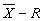control chart is used to keep the process centered.  If the process shifts off-target by 1.5s , the first point following the shift has a 70% chance of falling outside the control limits.  This control chart is capable of keeping the process within 1.5s of the target.  A process tolerance of 18 ± 0.15 £ 0.1 rpm could be specified.  This allows a ± 1.5operating window around the target.  In general, manually controlled processes are best represented by process tolerances specifying the operating window that can be maintained.  Stable processes and processes controlled using control charts typically hold a ± 1.5s operating window.

Example 4 – Line voltage (unstable process): The line voltage effects the seal strength of a sonic sealing machine. Voltage fluctuates between 120 ± 10 volts.  It cycles up and down. It can run for long periods of time in the upper end or the lower end of the range. This input is best described using the worst-case tolerance 120 ± 10 volts.  In general, unstable processes should be handled using worst-case or process tolerances.  If process tolerances are used, the minimum and maximum averages should represent the extremes seen over extended periods of time.

Example 5 – Sheeting Thickness (consistently off-target input):  Sheeting thickness affects seal strength of a heat sealer.  The requirements have been specified as 15 ± 1 mil (thousandth of an inch).  Suppose the sheeting supplier purposely runs below the target in order to reduce material costs.  They average 14.6 mils with a standard deviation of 0.2 mils. Off-center processes violate the assumption of statistical tolerancing.  Statistical tolerances should not be used is this case.  The process tolerance 15 ± 0.4 £0.2 mil accounts for the off-center average . In general, only process or worst-case tolerances should be used for consistently off-target processes.

Example 6 – 4-Component Stack (multiple identical components): In the 4-component stack problem, the 4-components are identical.  As a result, finished units consist of four components coming from the same supplier lot.  A capability study performed on the supplier’s process indicates that their process is in control with a standard deviation of 0.00667″.  Using historical data it is estimated that the process average varies from lot to lot with a standard deviation of 0.0075″.  The total standard deviation is then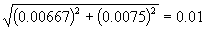.  However, specifying a statistical tolerance of 1.0 £0.01″ and performing a tolerance analysis can produce misleading results.  When the lot average is low, all the components tend to be low.  When the lot average is high, all the components tend to be high.  This creates a correlation between the inputs violating the assumption of independence.  Statistical tolerances should not be used is this case.  A process tolerance of 1.0 ± 0.015 £ 0.00667″ could be used instead.  It accounts for the effect of below and above average lots.  In general, only process and worst-case tolerances should be used for products containing multiple identical components or correlated inputs.

Example 7 – Spring Force (deterioration over time): The closure force of a car door hinge is affected by spring force.  The springs are purchased from a supplier.  The target is 10 lbs.  A capability study performed on the supplier’s process indicates that their process is in control with a standard deviation of 0.2 lbs.  A process tolerance of 10 ± 0.3 £ 0.2 lb. could be used, allowing a ± 1.5s operating window around the target.  This represents the manufacturing variation.  However, in this case, the springs are also prone to deterioration over time.  Life testing of the springs indicates that they deteriorate by 1 lb. over a 5 year period.  Deterioration is best represented by a worst-case tolerance since the closure force must be within specifications at both the beginning and the end of the 5 year period.  Deterioration is best represented by the worst-case tolerance 10 +0 –1 lb.  Combining these two tolerances results in 10 + 0.3 –1.3 £ 0.2 lb.  In general, deterioration of an input should be represented as a worst-case tolerance.  The deterioration effect should then be combined with the manufacturing variation to obtain the final tolerance.

SIX SIGMA QUALITY
There is an important link between six sigma quality and process tolerancing.  Six Sigma quality, a term introduced at Motorola, requires that a measurable characteristic such as flow rate or seal strength have no more the 3.4 defects per million units produced. This is in turn translated into the requirements that the average and standard deviation meet the conditions specified by the process tolerance ty ±D y £ s y where: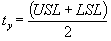(Eq. 32)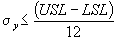(Eq. 33)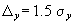(Eq. 34)

USL is the upper specification limit and LSL is the lower specification limit.  This process tolerance is shown in Figure 11.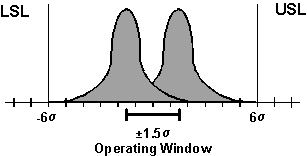Figure 11: Six Sigma Process Tolerance

For the process tolerance in Figure 11, the worst-case scenario is when the average is 1.5s off-target resulting in an average that is 4.5s from the nearest specification limit.  Based on the normal distribution, when the process average is 4.5s from the specification limit, the defect rate is 3.4 defects per million.  This is the definition of six sigma quality.  The name comes from the fact that six sigma quality requires specifications that are ± 6s wide.

Since the requirements for six sigma quality are specified in the form of a process tolerance, it is only natural that process tolerancing be used to ensure that these tolerances are achieved.  Process tolerancing provides an alternative to the combined dynamic and static mean tolerance analysis procedure described in Harry and Lawson (1992).

SUMMARY

Worst-case tolerancing tends to overestimate the variation of the output. Cost suffers when the variation of the output is overestimated.  Statistical tolerancing tends to underestimate the output variation. Quality suffers when the variation of the output is underestimated.  Using process tolerancing, it is possible to accurately predict the behavior of the output, providing the desired quality at a lower cost.

Process tolerancing provides a unified approach to tolerancing with encompasses both worst-case and statistical tolerancing.  It allows worst-case tolerances to be used for some inputs and statistical tolerance are used for others.  No longer does one have to use the same type of tolerance for all inputs.  The purpose of a tolerance analysis is to predict the behavior of the output based on knowledge of how the inputs behave. Key to its success is the ability to accurately describe the behavior of the inputs.  Using process tolerancing, you can describe behaviors for inputs not adequately described by either worst-case or statistical tolerances.  This provides solutions to commonly occurring problems such as off-center processes and multiple identical components.

REFERENCE LIST

Bender, A. (1962). Benderizing Tolerances – A Simple Practical Probability Method of Handling Tolerances for Limit-Stack-Ups. Graphic Science Dec.

Cox, N. D. (1986). How to Perform Statistical Tolerance Analysis. Milwaukee: ASQC Quality Press.

Evans, D. H. (1975). Statistical Tolerancing: The State of the Art. Journal of Quality Technology 7 (1): 1-12.

Harry, M. J. and Lawson J. R. (1992). Six Sigma Producibility Analysis and Process Characterization. New York: Addison-Wesley.

Taylor, W. A. (1991). Optimization and Variation Reduction in Quality. Libertyville, IL: Taylor Enterprises, Inc.

Taylor, W. A. (1997). VarTran User Manual. Libertyville, IL: Taylor Enterprises, Inc.

Presented at Fall Technical Conference, 1995

Scroll to Top